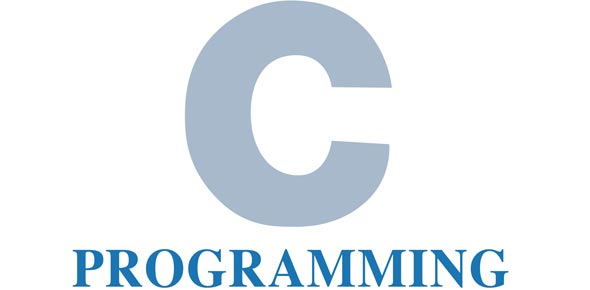# C Mock1

5 Questions | Total Attempts: 47SettingsIt is used to test the skill in c

Related Topics
• 1.
1.     What type of constant is 27U?
• A.

A. Short int constant

• B.

B. Character constant

• C.

C. Universal integer constant

• D.

D. Unsigned integer constant

• 2.
2.     The default  initial value of a static variable is _________
• A.

A. 0

• B.

B. 1

• C.

C. Default initial value as unpredictable value, which is often called a garbage value.

• D.

D. None from the list

• 3.
3.     Which one of the following is a valid variable name?
• A.

A. Account Number

• B.

B. "Account Number"

• C.

C. Account-Number

• D.

D. Account_Number

• 4.
4.     strcpy(name, "Anand");
• A.

A. The minimum size "name" could be is 5

• B.

B. The minimum size "name" could be is 6

• C.

C. "name" could be any size as it is resized after a copy

• D.

D. The parameters to strcpy are in the wrong order

• 5.
5.     When array name is passed to a function, what actually gets passed is
• A.